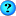#1
 VaqueroMember Join Date: Nov 2015 Posts: 62Custom Effect Shading

Hey!
I'm trying to change they way models get shaded, so I was looking into the custom effects. The documentation only gives very simple examples.

When things get more complicated I need helper functions, but I suspect these are not supported to work with vizard's effects framework?
Let's do a really simple dummy example.

Assume inside the shader code I want to use this helper function:
Code:
```float halve(float k)
{
return k*0.5;
}```
Of course, in a real world example it will be much more complicated than this.

But whereever I put this function, it throws some error. Inside Shader, but outside Units:
Code:
```# Setup model

code = """

float halve(float k)
{
return k*0.5;
}

BEGIN FinalColor
float half_r = halve(gl_FragColor.r);
gl_FragColor.rgb = vec3(half_r, 1.0, 1.0);
END
}
}
"""